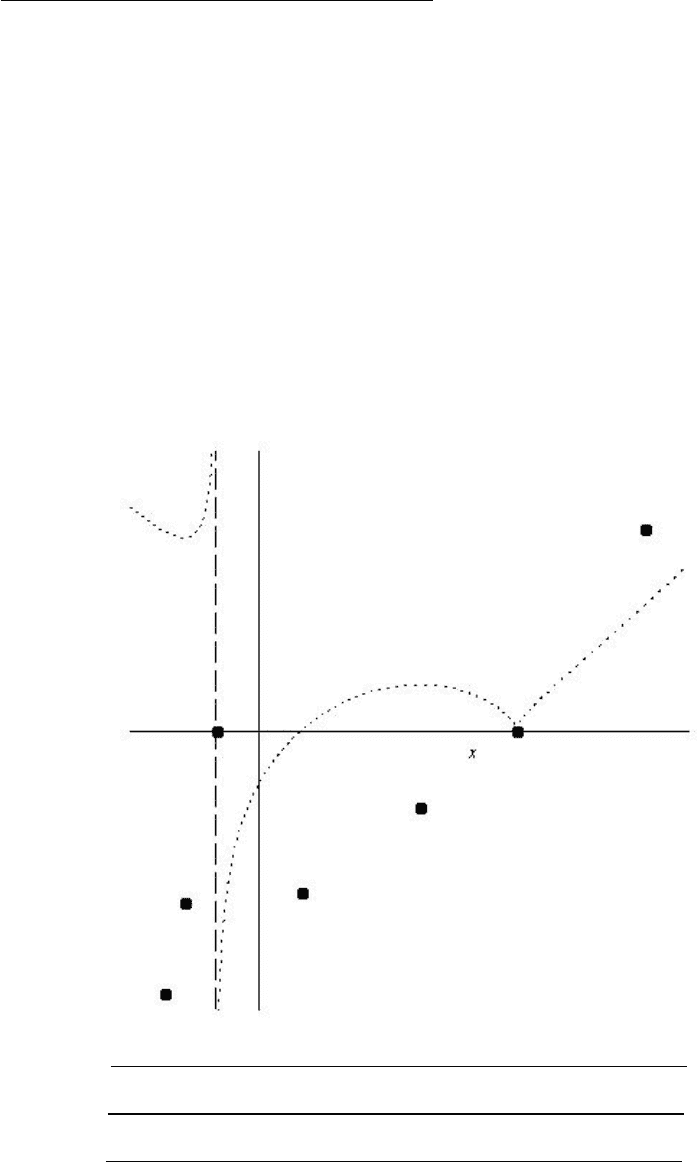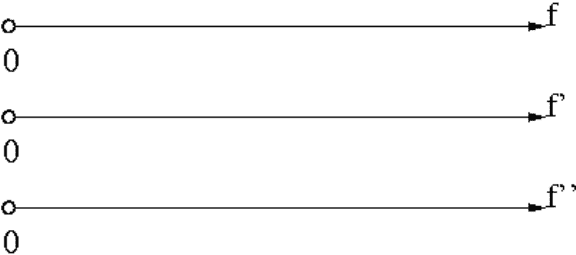# MTH 151 Midterm: MTH151 Term Test 2 2009 Fall

53 views7 pagesName:
Exam 2 - Math 151
Oct 30, 2009
R. Ketchersid
1. [10pts] The following is a graph of f0(x)for some function f(x)which is continuous on R. Some points
which lie on the graph of f(x)are indicated.
Fill out the sign diagrams for f(x),f0(x), and f00 (x). (Indicate the domains correctly.)
Sketch the graph of f(x).
Indicate the points of inﬂection by drawing a circle around them.
Indicate the local extrema by drawing a box around them.
Make your sign diagrams line up with the graph, if it does not, then I will not give you credit. I want this
part to be neat and easy to grade.
f
f0
f00
Unlock document

This preview shows pages 1-2 of the document.
Unlock all 7 pages and 3 million more documents.-2-
2. [10pts] Consider the function
f(x) = xln(x)
(a) Find f0(x)and f00 (x)and simplify enough to ﬁnd their roots.
(b) Complete the sign diagrams for f(x),f0(x), and f00 (x). (Make sure they line up.)
(c) At what values of xdoes f(x)have a local min/max?
(d) At what values of xdoes a point of inﬂection occur?
(e) What is limx0+f(x)?
Unlock document

This preview shows pages 1-2 of the document.
Unlock all 7 pages and 3 million more documents.

## Document Summary

Make your sign diagrams line up with the graph, if it does not, then i will not give you credit. I want this part to be neat and easy to grade. f f(cid:48) f(cid:48)(cid:48: [10pts] consider the function. 3 : [10pts] a fence 8 ft tall runs parallel to a tall building at a distance of 4 ft from the building. What is the length of the shortest ladder that will reach from the ground over the fence to the wall of the building? (i have supplied a diagram that suggests using as the independent variable. This is not the only way to do the problem; you do not have to follow my hint. If you use , then when you nd the critical point you will. Nd using an inverse trig function, for example, if i want so that sin5( ) = 4, then i take = sin 1(41/5)